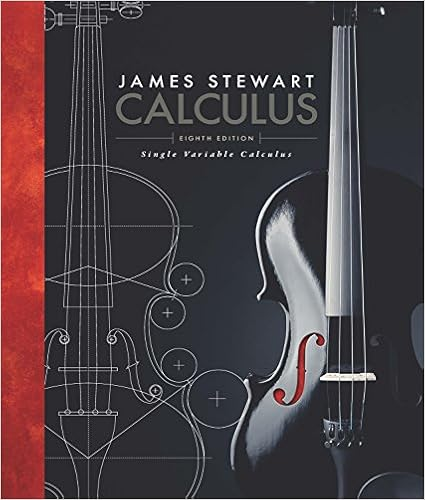# 8 Find the area of the region enclosed by the given...

• phlexmpoloka
• 2

This preview shows page 1 - 2 out of 2 pages.

##### We have textbook solutions for you!
The document you are viewing contains questions related to this textbook.The document you are viewing contains questions related to this textbook.
Chapter 8 / Exercise 8
Single Variable Calculus
StewartExpert Verified
Math 141, Section 04 & 05, Integral Calculus Exam 2(Self Evaluation Test 1): March 6, 2010 (100 points) Last name : First name : Student No. : Instructions : There are 8 questions on a total of 7 pages, including this cover page. Write your solutions in the space provided. Read each question carefully and show all your work . Provide exact answers (instead of decimal approximations) unless indicated otherwise. Only Sharp EL531M, EL531Q, EL509VHB, EL531VB, and Texas Instruments TI- 32, TI-32-solar, TI-30X, TI-30XA (solar or battery), TI30X-IIS, and TI30X-IIB are permitted. Question Points Score 1 8 2 24 3 12 4 8 5 8 6 8 7 8 8 8 Total: 84
##### We have textbook solutions for you!
The document you are viewing contains questions related to this textbook.The document you are viewing contains questions related to this textbook.
Chapter 8 / Exercise 8
Single Variable Calculus
StewartExpert Verified
1. (8) Find the area of the region enclosed by the given curves. y = e - x sin x , y = - e - x sin x , 0 x 2 π
2. (24) Find the volume of the solid obtained by rotating the region bounded by the given curves about the specified line (use both disk method and shell method if possible). 1. y = x 3 , y = x 2 about the y
•••